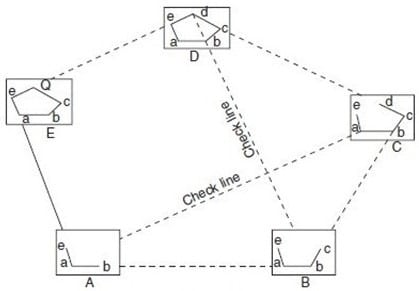# Plane Table Surveying on Civil Engineering site

Table of Contents

# Plane Table Surveying

Plane table is surveying the method of surveying by which the position of all the present object on the plane field is determined by simple observation, and plotted on a sheet. In this distance is measured by tape & chain and the angles are measured by compass or theodolite.

Working Operation of A Plane Table

1. Levelling
2. Centering
3. Orientation

## Method of Plane Table Surveying

Following methods are employed for surveying with a plane table

1. Radiation Method
2. Intersection Method
3. Traversing Method
4. Resection Method

### Radiation

This method is suitable for small distance surveying, usually the distance which covered in a single distance.

In this method, we place plane table (Johnson Table 45×60 cm) on plane ground whose center is denoted as “O” as shown in fig. From point O, sight the points A,B,C,D and E using Alidade, and locate the points and plot the points as a,b,c,d and e in the drawing sheet.

###Intersection

This method is also called Graphical Triangulation.

It is mostly suitable in hilly area.

In this method locate the point by plotting two lines from two known points it form a triangle in plotting. As shown in figure, P and Q are the known points. First the equipment is placed on P and plot the lines by sighting the stations A, B and Q. then shift the equipment to point Q and plot the lines by sighting stations A, B and P.### Traversing

Traversing is the connection of series of straight lines. In case of traversing, plane table is located at one point for suppose A as shown below. From that point sight towards B and measure the distance AB. Then shift the plane table to point B and sight towards A and measure BA. Average distance of AB and Ba are plotted to scale in drawing sheet. Then Sight the point C from B and measure BC and repeat the same procedure until last point. Conduct some checks at some points. Finally traverse lines are plotted on the drawing sheet.### Resection

Resection is a method of plane table surveying in which the location of plane table is determined by sighting it from known points or plotted points. It is also called method of orientation and resection can be done by following methods as follows.

### Two Point Problem:

Finding the location of plane table on the paper, by sighting from two points whose locations have previously been plotted on the paper, is known as Two Point Problem.

### Three Point Problem:

Locate and determine the position of plane table from our previously defined three points and plot on sheet of plane table is known as three point problem.

#### Three point problem solved by one of the following methods:

1. Mechanical (or Tracing Paper) Method
2. Bessel’s Graphical Method
3. Method of Perpendiculars
4. Trial and Error Method ( Or Lehman’s Method)